# 🌈欢迎来到C++专栏~~Map 和Set

• (꒪ꇴ꒪(꒪ꇴ꒪ )🐣,我是Scort
• 目前状态：大三非科班啃C++中
• 🌍博客主页：张小姐的猫~江湖背景
• 快上车🚘，握好方向盘跟我有一起打天下嘞！
• 送给自己的一句鸡汤🤔：
• 🔥真正的大师永远怀着一颗学徒的心
• 作者水平很有限，如果发现错误，可在评论区指正，感谢🙏
• 🎉🎉欢迎持续关注！## 一. 关联式容器

forward_list(C++11)等，这些容器统称为序列式容器，因为其底层为线性序列的数据结构，里面存储的是元素本身。那什么是关联式容器？它与序列式容器有什么区别？

set、map、multiset、multimap树型结构平衡搜索树（红黑树）
unordered_set、unordered_map、unordered_multiset、unordered_multimap哈希结构哈希表，哈希桶

## 二. 键值对

• 现在要建立一个英汉互译的字典，那该字典中必然有英文单词与其对应的中文含义，而且，英文单词与其中文含义是一一对应的关系，即通过该应该单词，在词典中就可以找到与其对应的中文含义

SGI-STL中关于键值对pair的定义

template <class T1, class T2>
struct pair
{
typedef T1 first_type;
typedef T2 second_type;
T1 first;
T2 second;

pair(): first(T1()), second(T2())
{}
pair(const T1& a, const T2& b): first(a), second(b)
{}
};


## 三. C++中的Set

### 1️⃣Set的介绍

Set本质就是Key的模型，就是确认该值在不在

### 2️⃣Set的使用（参照文档）

Set支持的操作是增删查，不能改！（因为底层是一颗搜索树，改了就乱了）

#### 🌈set的模板参数列表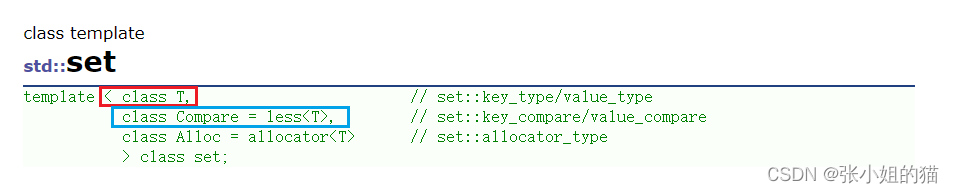• T：set中存放元素的类型，实际在底层存储<value, value>的键值对
• Compare仿函数；set中元素默认按照小于来比较（如果比较的方式不满意，可以自己去控制比较规则：自己写仿函数）
• Alloc：set中元素空间的管理方式，使用STL提供的空间配置器内存池

#### 🌈set的构造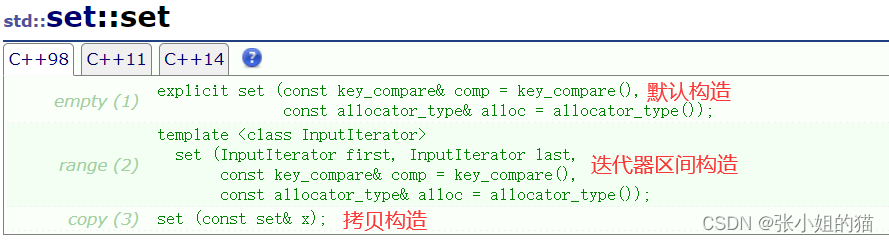void testset2()
{
set<int> set1;//空构造
int num[] = { 1, 2, 3, 7, 9, 3, 10, 1 };

//区间构造
set<int> set2(num, num + sizeof(num) / sizeof(num));

//拷贝构造
set<int> set3(set2);

for (auto& e : set3) //打印结果看出set可以去重
{
cout << e << " ";
}
cout << endl;
}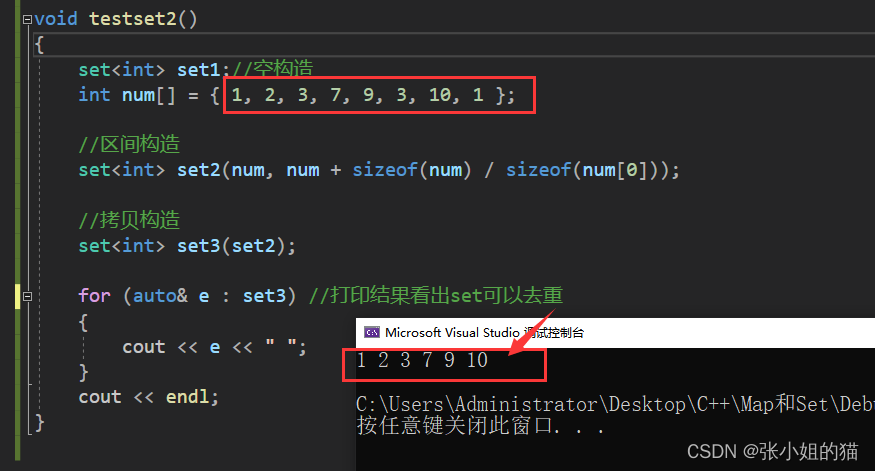#### 🌈set的迭代器

begin + end（重点）获取第一个数据位置的， 获取最后一个数据下一个位置
cbegin() const 和 cend（）const无非就是针对const的版本，不可写
rbegin + rend获取最后一个数据位置，获取第一个数据前一个位置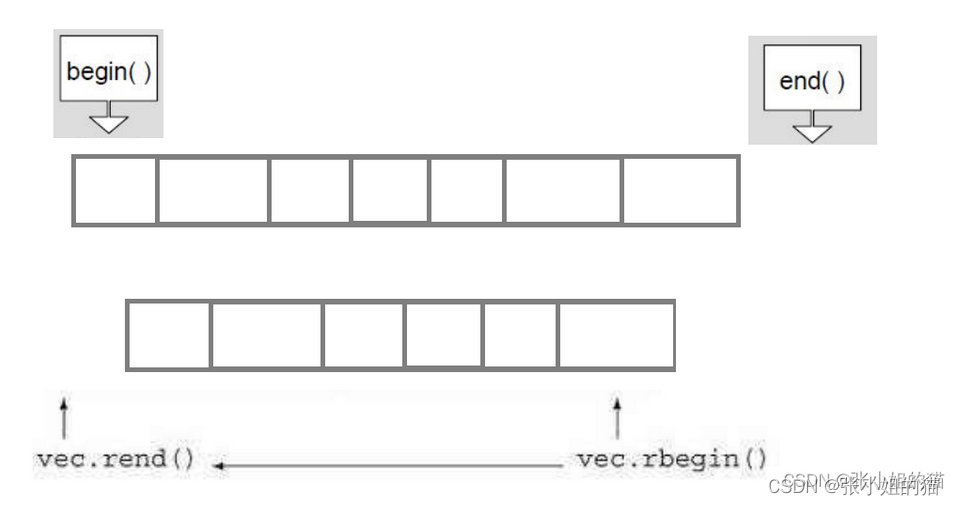void test_set()
{
//set<int> s;
//s.insert(1);
//s.insert(2);
//s.insert(3);

set<int> s = { 1, 2, 1, 6, 3, 8, 5 };
//排序 + 去重
set<int>::iterator it = s.begin();
while (it != s.end())
{
cout << *it << " ";
++it;
}
cout << endl;

//支持迭代器也就支持范围for了
for (auto e : s)
{
cout << e << " ";
}
cout << endl;
}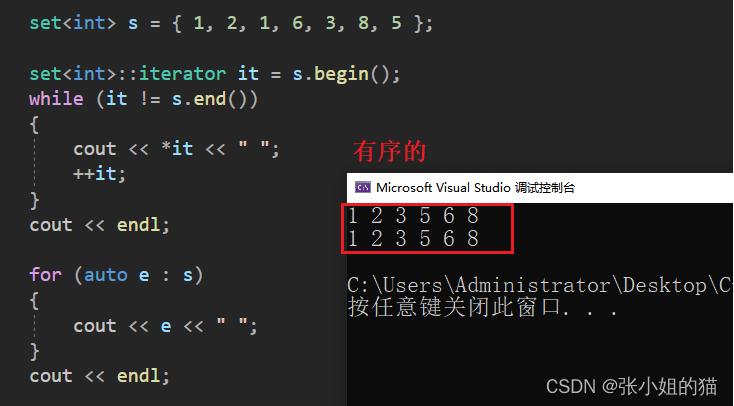1. 反向迭代器
2. 仿函数： less—>greater
	int a[] = { 1, 2, 1, 6, 3, 8, 5 };
set<int, greater<int>> s(a, a + sizeof(a) / sizeof(int));


#### 🌈set的常见修改操作

🥑find & erase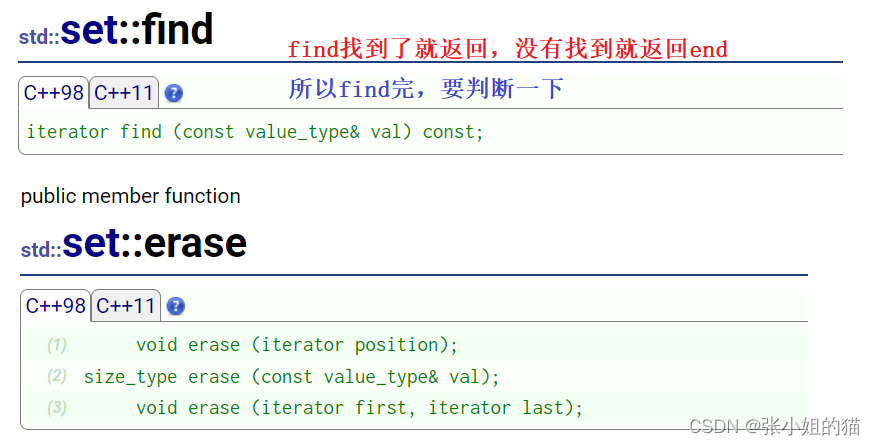• 我删除一个不存在的值呢？会怎么样？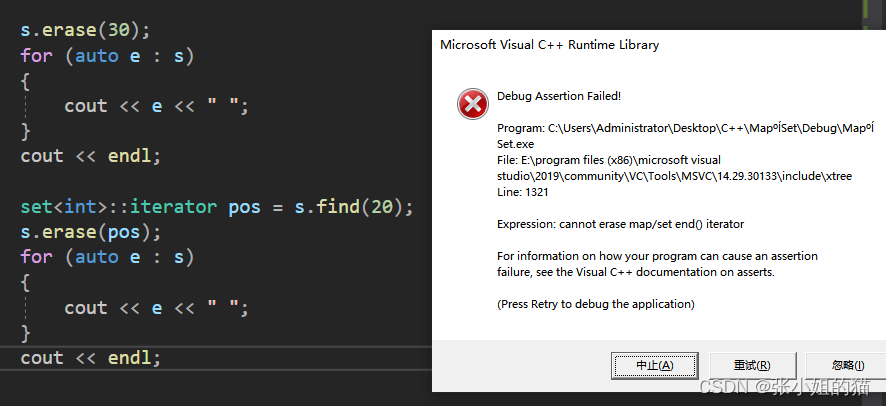set<int>::iterator pos = s.find(20);
if (pos != s.end())
{
s.erase(pos);
}


🥑count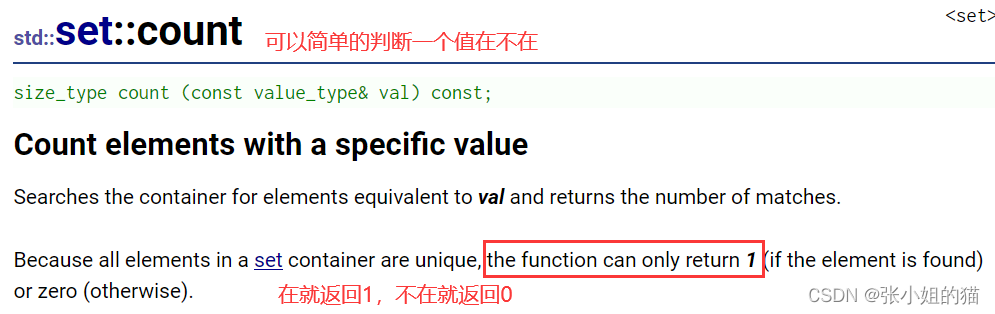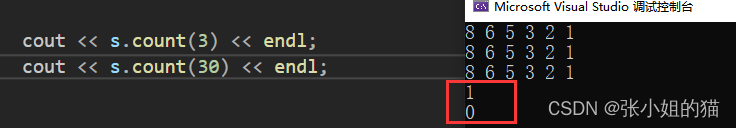🥑lower_bound 和 upper_bound

• lower_bound下限的意思，返回 >= val的值
• upper_bound上限的意思，返回 > val的值
	itlow = myset.lower_bound(35);//返回大于等于35的值
itup = myset.upper_bound(60);//返回大于60的值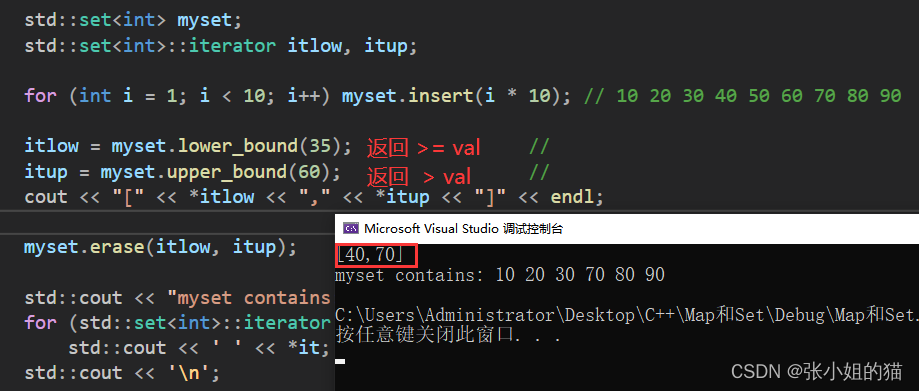### 3️⃣Multiset的用法

multiset容器与set容器实现和接口基本一致，唯一区别就是，multiset 允许键值冗余，即multiset容器当中存储的元素是可以重复的

void testset4()
{
int a[] = { 1, 2, 1, 6, 3, 8, 5 };
//允许键值冗余
multiset<int> s(a, a + sizeof(a) / sizeof(int));

for (auto e : s)
{
cout << e << " ";
}
cout << endl;
}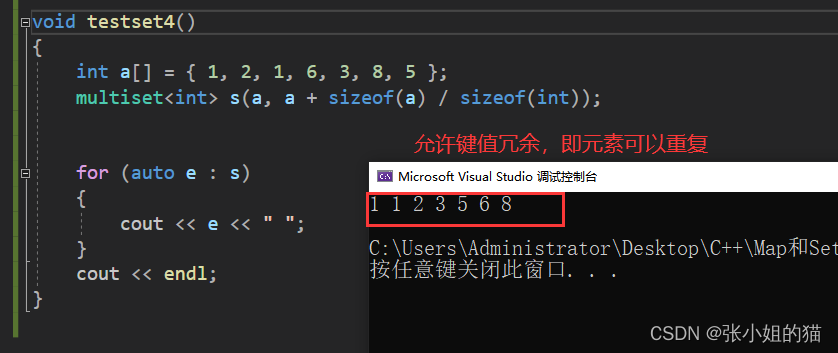1. find时，如果有多个值，返回中序的第一个
void testset4()
{
int a[] = { 1, 2, 1, 6, 3, 8, 5, 3};
//允许键值冗余
multiset<int> s(a, a + sizeof(a) / sizeof(int));

//find时，如果有多个值，返回中序的第一个
auto pos = s.find(3);
while (pos != s.end())
{
cout << *pos << " ";
++pos;//这里的++ ，就是加到下一个的3的位置
}
cout << endl;
}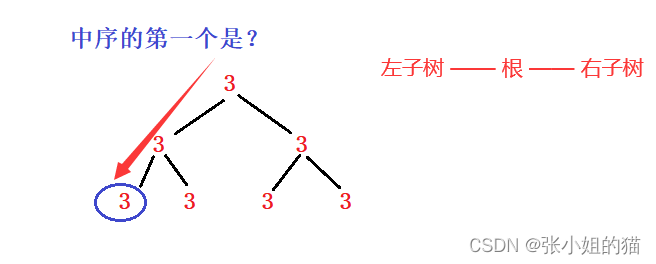## 四. C++中的Map

### ⚡Map的介绍

1. map是关联容器，它按照特定的次序(按照key来比较)存储由键值key和值value组合而成的元素。
2. 在map中，键值key通常用于排序和惟一地标识元素，而值value中存储与此键值key关联的内容。键值key和值value的类型可能不同，并且在map的内部，key与value通过成员类型value_type绑定在一起，为其取别名称为pair: typedef pair<const key, T> value_type;
3. 在内部，map中的元素总是按照键值key进行比较排序的。
4. map中通过键值访问单个元素的速度通常比unordered_map容器慢，但map允许根据顺序对元素进行直接迭代(即对map中的元素进行迭代时，可以得到一个有序的序列)。
5. map支持下标访问符，即在[]中放入key，就可以找到与key对应的value
6. map通常被实现为二叉搜索树(更准确的说：平衡二叉搜索树(红黑树))。

### ⚡Map的用法

#### 💦Map的模板参数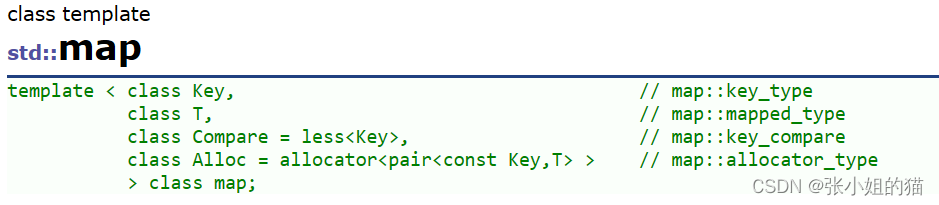1. key: 键值对中key的类型

2. T： 键值对中value的类型

3. Compare: 比较器的类型，map中的元素是按照key来比较的，缺省情况下按照小于来比较，一般情况下(内置类型元素)该参数不需要传递，如果无法比较时(自定义类型)，需要用户自己显式传递比较规则(一般情况下按照函数指针或者仿函数来传递)

4. Alloc：通过空间配置器来申请底层空间，不需要用户传递，除非用户不想使用标准库提供的空间配置器

#### 💦Map的迭代器

	//map<string, string>::iterator it = dict.begin();
auto it = dict.begin();

while (it != dict.end())
{
//pair没有重载流插入
//cout << (*it).first << (*it).second << endl;
//cout << it->->first << it->->second << endl;
//operator重载
cout << it->first << it->second << endl;

++it;
}

for (const auto& kv : dict)//给const & 引用
{
cout << kv.first << ":" << kv.second << endl;
}
cout << endl;


#### 💦Map的构造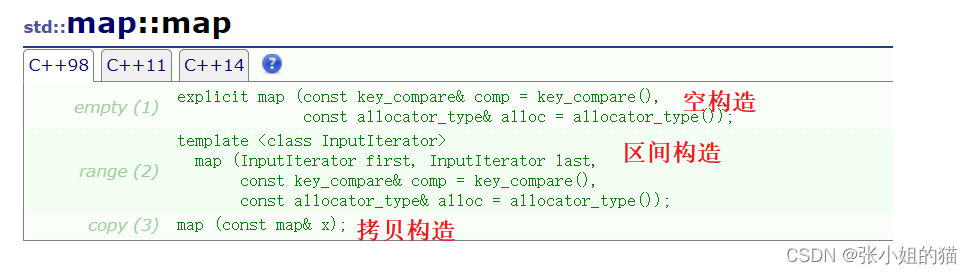void testmap1()
{
map<int, int> map1;//空构造
int num[] = { 1,5,9,4,8,2,3,1,5,4,5,7 };
for (auto e : num)
{
map1.insert(make_pair(e,e));
}
map<int, int> map2(map1.begin(),map1.end());//迭代区间构造
map<int, int> map3(map2);//拷贝构造
for (auto& e : map3)
{
cout << e.first << ":" << e.second << endl;
}
}


#### 💦Map的常见修改操作

🌏Insert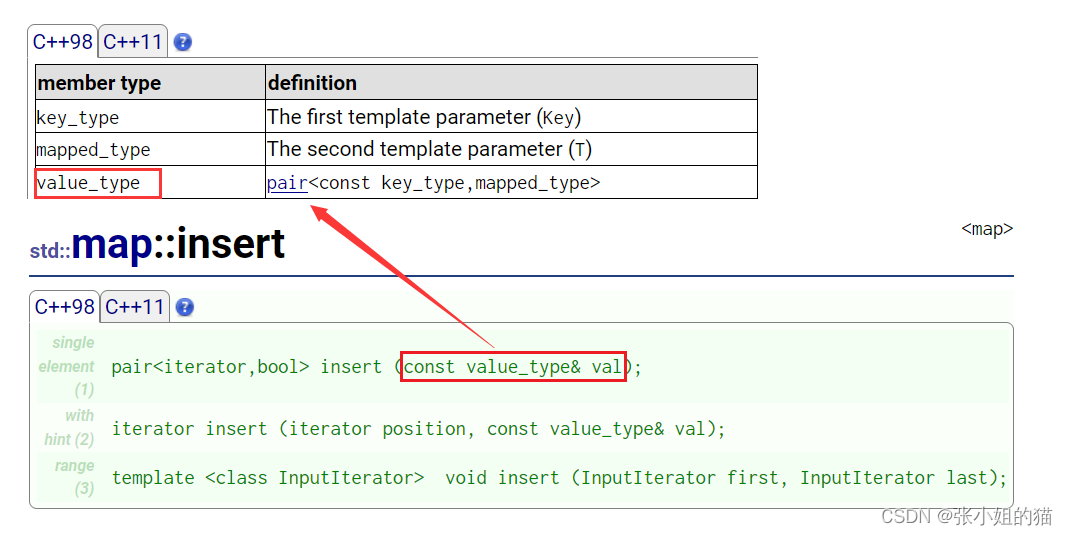find插入的是pair的结构体，此版本是不支持冗余的，插入只看key值，有相等的就不需要了

void test_map1()
{
map<string, string> dict;
//pair<string, string> kv1("sort", "排序");
//dict.insert(kv1);

//匿名对象
dict.insert(pair<string, string>("sort", "排序"));
dict.insert(pair<string, string>("test", "测试"));
dict.insert(pair<string, string>("string", "字符串"));

//宏替换
typedef pair<string, string> Dictkv;
dict.insert(Dictkv("string", "字符串"));
}


	dict.insert(make_pair("string", "字符串"));//很常用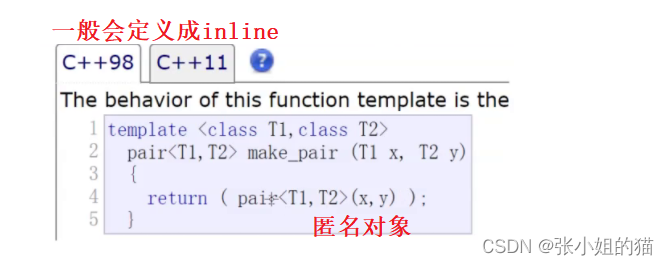#### 💦Map的[ ]的使用（重头戏）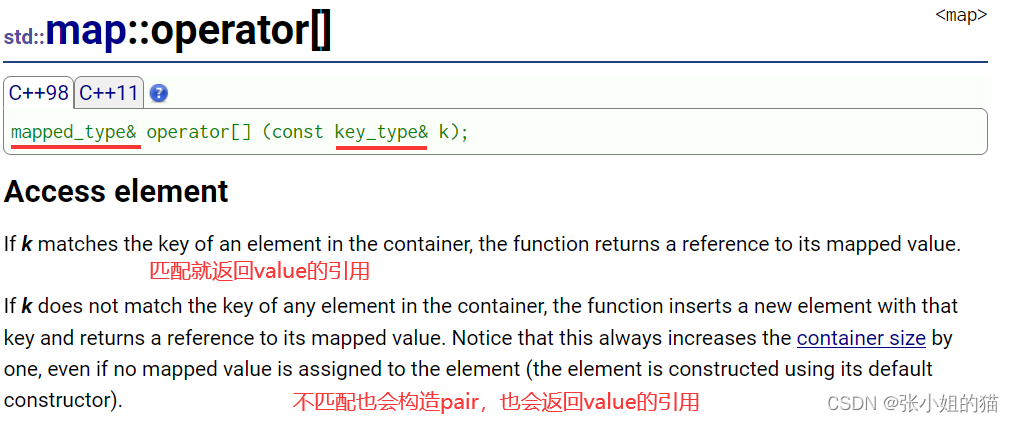[]:的功能：查找 + 修改

• map中有这个key，就返回value引用（查找 + 修改）
• map中没有这个key，会插入一个pair(key, K())，会插入这个key值，value就要去调用默认构造，最后还是返回value的引用 （插入+ 修改）

	map<string, string> dict;
dict.insert(make_pair("sort", "排序"));
dict["insert"];                       //插入
dict["insert"] = "插入";              //插入 + 修改value返回值
dict["left"] = "左边";                //插入 + 修改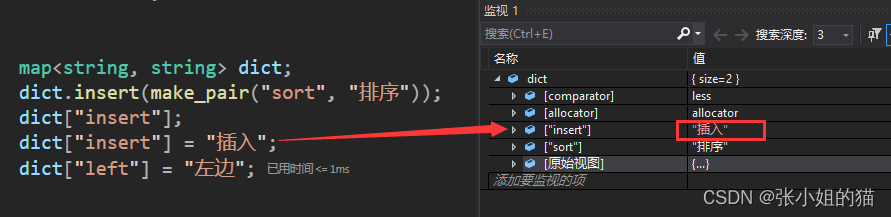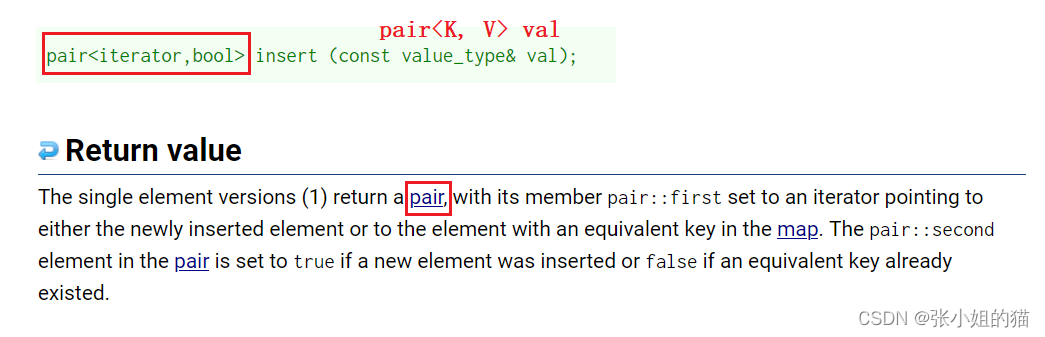• key已经在map中，返回pair（key_iteratorfalse
• key不在map中，返回pair（new_key_iteratortrue

[]其底层实现：有两个pair结构

• ✨返回值是一个pair结构pair<iterator, bool>；第一个值是迭代器，第二个值是bool；第二个bool是用来反应是否插入成功，如果成功，则 第一个值迭代器就是指向插入的pair数据
• 第二个是kv的pair,是插入的pair数据
V& operator[](const K& key)
{
pair<iterator, bool> ret = insert(make_pair(key, V());
//返回key节点的迭代器，迭代器是map的，再调用->就是pair*,取second
return ret.first->second;
}


### ⚡统计次数的两种方法

1. 第一次找不到的时候make_pair，使得count = 1
2. 后面每遍历一次就++一次
//统计次数
void test_map2()
{
string arr[] = { "苹果", "西瓜", "苹果", "西瓜", "苹果", "苹果", "西瓜","苹果", "香蕉", "苹果", "香蕉" };
map<string, int> countMap;
for (auto& str : arr)
{
map<string, int>::iterator it = countMap.find(str);
if (it != countMap.end())
{
/*(*it).second++;*/
//it->->second++;  第一个箭头是调用operator->返回的是pair*，再->指向成员
it->second++;
}
else
{
//找不到
countMap.insert(make_pair(str, 1));
}
}

//遍历
map<string, int>::iterator it = countMap.begin();
while(it != countMap.end())
{
cout << it->first << ":" << it->second << endl;
++it;
}
cout << endl;
}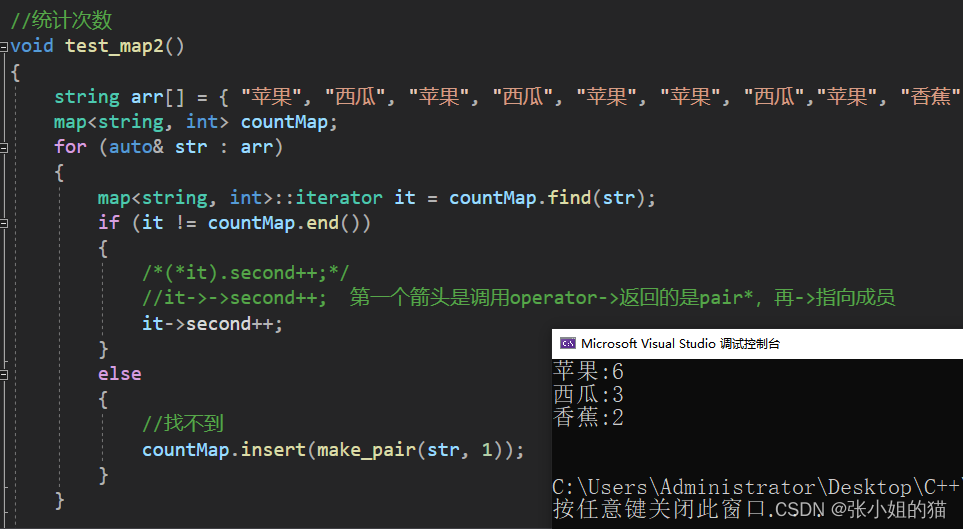map<string, int> countMap;
for (auto& str : arr)
{
//1、str不在countMap中，插入pair(str, int()),然后对返回次数++
//2、str在的话，就返回value的引用次数++
countMap[str]++;
}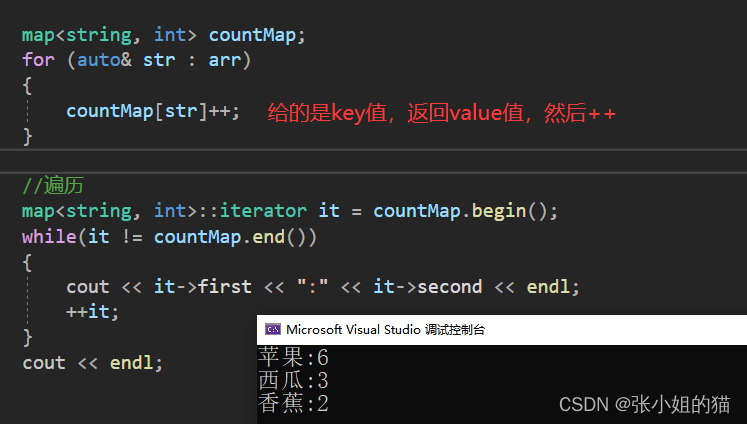### ⚡multimap的用法

multimap容器与map容器的底层实现以及成员函数的接口都是基本一致，区别是multimap 允许键值冗余，即multimap容器当中存储的元素是可以重复的

	multimap<string, string> mdict;
mdict.insert(make_pair("sort", "排序"));
mdict.insert(make_pair("left", "左边"));
mdict.insert(make_pair("left", "左边"));
mdict.insert(make_pair("left", "你猜"));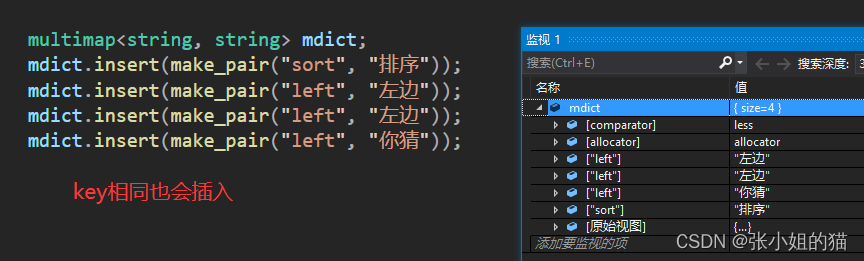## 五. 来两道题目练练手

### 1️⃣前K个高频单词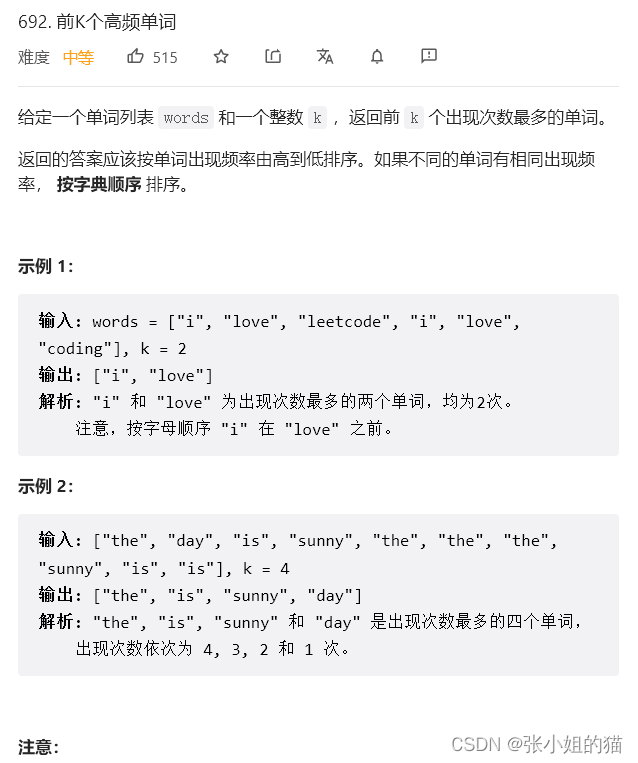1. 使用一个countMap统计各种单词的出现的次数（统计次数 此时是i在上面，love在下面）
2. 再写一个sortMap按照字符出现的次数进行降序排列
3. 不同的单词出现相同的次数，会按字典序排列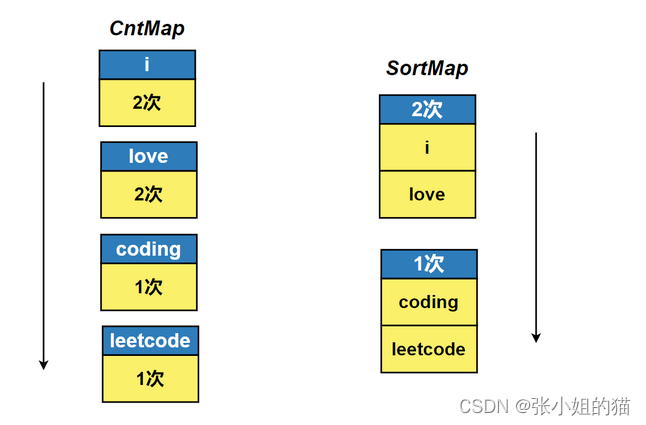class Solution {
public:
vector<string> topKFrequent(vector<string>& words, int k) {
map<string, int> countMap;
//统计次数   此时是i在上面，love在下面
for(auto& str : words)
{
countMap[str]++;
}

// apple 3  \  i  2  \  love  2 \ j  5
multimap<int, string, greater<int>> sortMap;//排降序
for(auto& kv : countMap)
{
sortMap.insert(make_pair(kv.second, kv.first));
}

vector<string> v;
multimap<int, string, greater<int>> :: iterator it = sortMap.begin();

for(size_t i = 0; i < k; i++)
{
v.push_back(it->second);
it++;
}
return v;
}
};


### 2️⃣两个数组的交集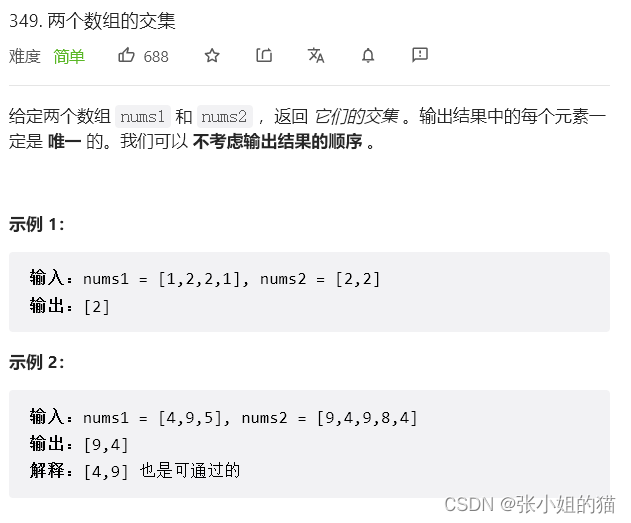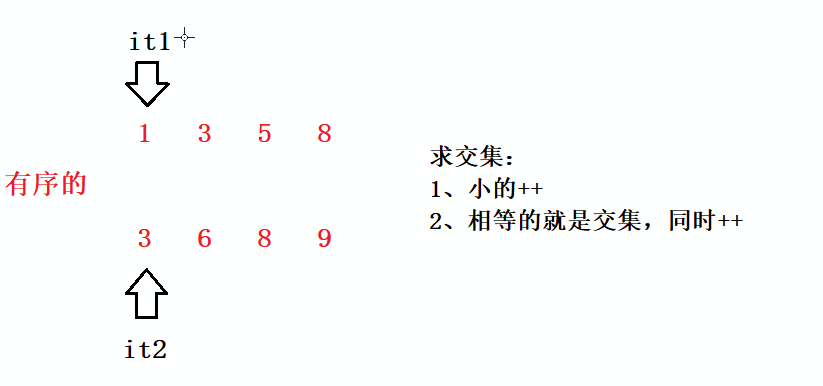class Solution {
public:
vector<int> intersection(vector<int>& nums1, vector<int>& nums2) {
set<int> s1 (nums1.begin(), nums1.end());
set<int> s2 (nums2.begin(), nums2.end());

set<int> :: iterator it1 = s1.begin();
auto it2 = s2.begin();

vector<int> v;
while(it1 != s1.end() && it2 != s2.end())
{
if(*it1 < *it2)
{
++it1;
}
else if(*it1 > *it2)
{
++it2;
}
else
{
v.push_back(*it1);
++it1;
++it2;
}
}
return v;
}
};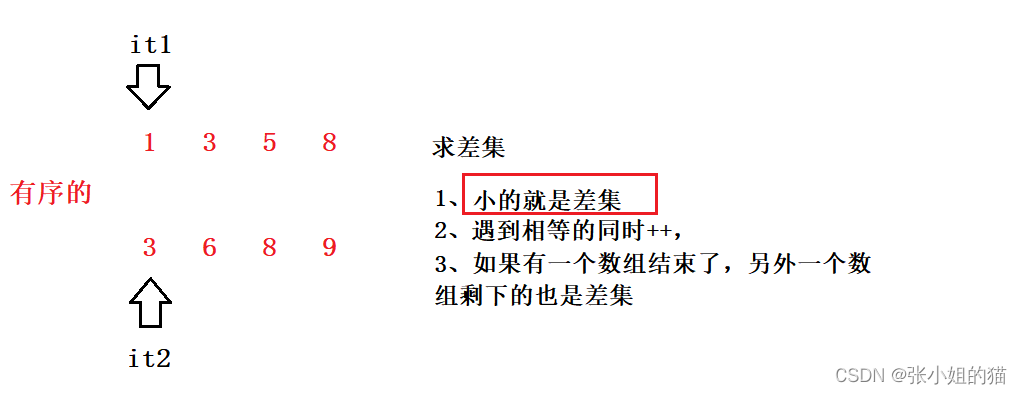# 📢写在最后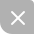UP更新不错过~
09-20
09-16
09-17
10-14
08-26
09-19
05-23416

### “相关推荐”对你有帮助么？

•非常没帮助
•没帮助
•一般
•有帮助
•非常有帮助被折叠的  条评论 为什么被折叠?到【灌水乐园】发言¥2 ¥4 ¥6 ¥10 ¥20余额支付 (余额：-- )扫码支付获取中扫码支付点击重新获取扫码支付1.余额是钱包充值的虚拟货币，按照1:1的比例进行支付金额的抵扣。
2.余额无法直接购买下载，可以购买VIP、C币套餐、付费专栏及课程。余额充值